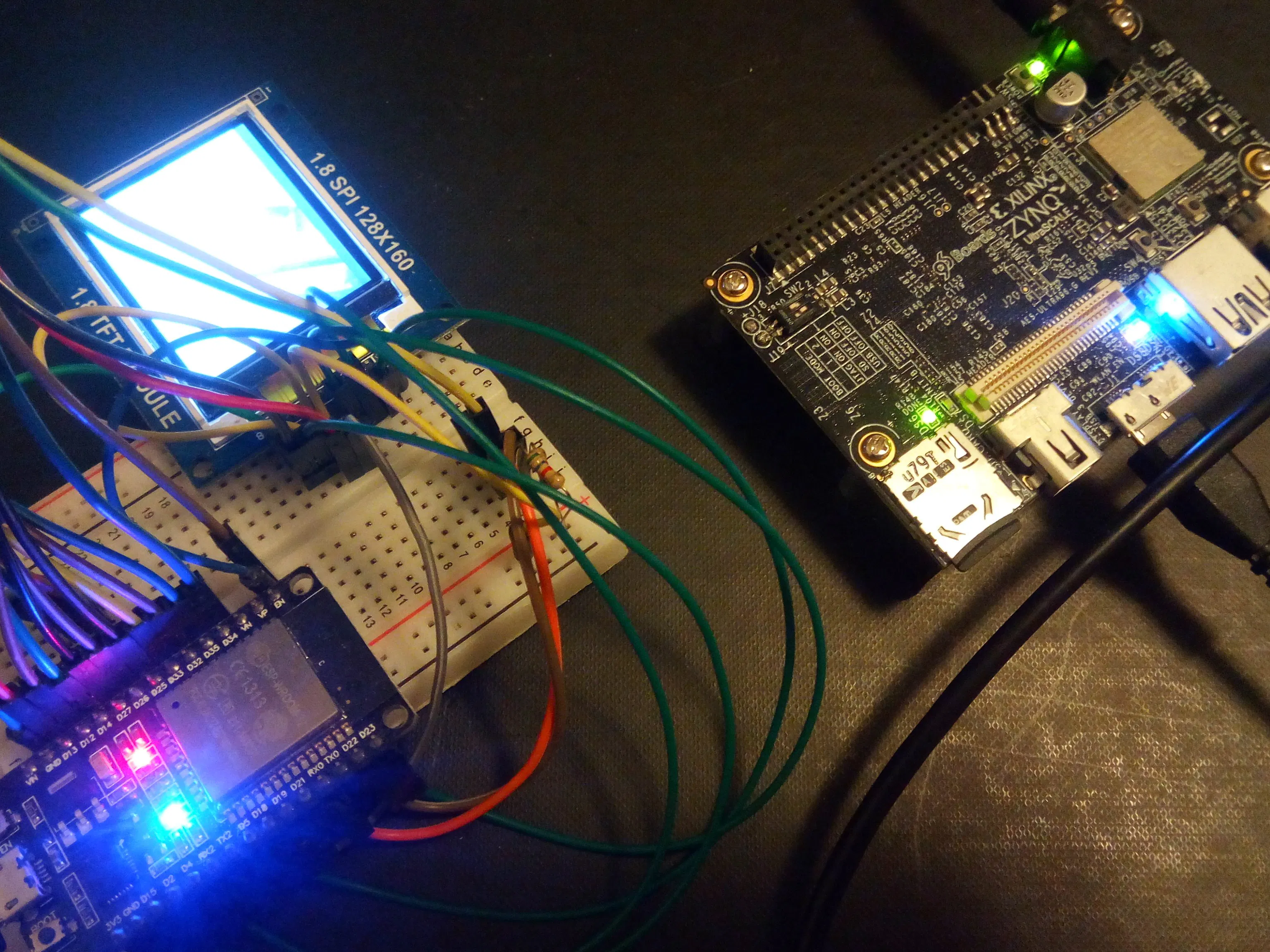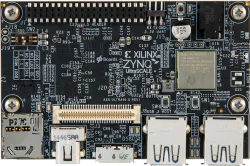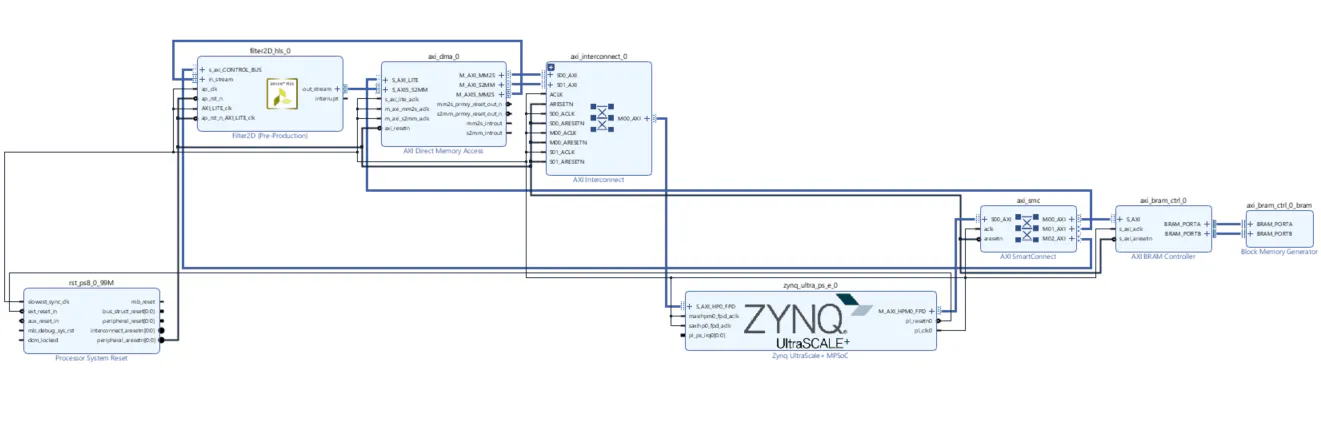# Accelerating Image Processing with Ultra96

Use a ZYNQ UltraScale+ to build a distributed camera vision system with image processing capabilities.## Things used in this project

### Hardware componentsAvnet Ultra96
×1

### Software apps and online servicesPYNQ V2.3

## Schematics

### Custom Filter HDL IP

block diagram on Vivado 2018.3 of the convolutional IP core.### Filter2D block diagram## Code

### Filter2D_PYNQ_overlay_notebook

Python
Python Jupyter notebook for Convolutional Filter2D IP core
```# coding: utf-8
#
# 2D convolutional filter PYNQ overlay
# Copyright (C) February 16 2019  dhq

# This program is free software: you can redistribute it and/or modify
# the Free Software Foundation, either version 3 of the License, or
# (at your option) any later version.

# This program is distributed in the hope that it will be useful,
# but WITHOUT ANY WARRANTY; without even the implied warranty of
# MERCHANTABILITY or FITNESS FOR A PARTICULAR PURPOSE.  See the
# GNU General Public License for more details.

# You should have received a copy of the GNU General Public License
# along with this program.  If not, see <http://www.gnu.org/licenses/>.

# In:

import os
import numpy as np
from pynq import Overlay, PL, MMIO
from pynq import DefaultIP, DefaultHierarchy
from pynq import Xlnk
from pynq.xlnk import ContiguousArray
from pynq.lib import DMA
from cffi import FFI

CV2PYNQ_ROOT_DIR = os.path.dirname(os.path.realpath('__file__'))

class cv2pynq():
MAX_WIDTH  = 800
MAX_HEIGHT = 600
self.bitstream_name = None
self.bitstream_name = "imgfilter.bit"
self.bitstream_path = os.path.join(self.bitstream_name)
self.ol = Overlay(self.bitstream_path)
self.ol.reset()
self.xlnk = Xlnk()
self.partitions = 10 #split the cma into partitions for pipelined transfer
self.cmaPartitionLen = self.MAX_HEIGHT*self.MAX_WIDTH/self.partitions
self.listOfcma = [self.xlnk.cma_array(shape=(int(self.MAX_HEIGHT/self.partitions),self.MAX_WIDTH), dtype=np.uint8) for i in range(self.partitions)]
self.filter2d = self.ol
self.dmaOut = self.filter2d.axi_dma_0.sendchannel
self.dmaIn =  self.filter2d.axi_dma_0.recvchannel
self.dmaOut.stop()
self.dmaIn.stop()
self.dmaIn.start()
self.dmaOut.start()
self.filter2DType = -1  # filter types: SobelX=0
self.ffi = FFI()
self.f2D = self.filter2d.filter2D_hls_0
self.f2D.reset()
self.cmaBuffer_0 = self.xlnk.cma_array(shape=(self.MAX_HEIGHT,self.MAX_WIDTH), dtype=np.uint8)
self.cmaBuffer0 =  self.cmaBuffer_0.view(self.ContiguousArrayCv2pynq)
self.cmaBuffer0.init(self.cmaBuffer_0)
self.cmaBuffer_1 = self.xlnk.cma_array(shape=(self.MAX_HEIGHT,self.MAX_WIDTH), dtype=np.uint8)
self.cmaBuffer1 =  self.cmaBuffer_1.view(self.ContiguousArrayCv2pynq)
self.cmaBuffer1.init(self.cmaBuffer_1)
self.cmaBuffer_2 = self.xlnk.cma_array(shape=(self.MAX_HEIGHT*4,self.MAX_WIDTH), dtype=np.uint8) # *4 for CornerHarris return
self.cmaBuffer2 =  self.cmaBuffer_2.view(self.ContiguousArrayCv2pynq)
self.cmaBuffer2.init(self.cmaBuffer_2)

def close(self):
self.cmaBuffer_0.close()
self.cmaBuffer_1.close()
self.cmaBuffer_2.close()
for cma in self.listOfcma:
cma.close()

def copyNto(self,dst,src,N):
dstPtr = self.ffi.cast("uint8_t *", self.ffi.from_buffer(dst))
srcPtr = self.ffi.cast("uint8_t *", self.ffi.from_buffer(src))
self.ffi.memmove(dstPtr, srcPtr, N)

def copyNtoOff(self,dst,src,N,dstOffset,srcOffset):
dstPtr = self.ffi.cast("uint8_t *", self.ffi.from_buffer(dst))
srcPtr = self.ffi.cast("uint8_t *", self.ffi.from_buffer(src))
dstPtr += dstOffset
srcPtr += srcOffset
self.ffi.memmove(dstPtr, srcPtr, N)

def filter2D(self, src, dst):
if dst is None :
self.cmaBuffer1.nbytes = src.nbytes
self.dmaIn.transfer(dst)
self.dmaOut.transfer(src)
self.dmaIn.wait()
return dst
self.dmaIn.transfer(self.cmaBuffer1)
self.dmaOut.transfer(src)
self.dmaIn.wait()
else:#pipeline the copy to contiguous memory and filter calculation in hardware
if src.nbytes < 184800: #440x420
self.partitions = 1
elif src.nbytes < 180000: #600x300
self.partitions = 2
elif src.nbytes < 231200: #680x340
self.partitions = 4
else :
self.partitions = 8
self.cmaBuffer1.nbytes = src.nbytes
self.dmaIn.transfer(self.cmaBuffer1)
chunks_len = int(src.nbytes / (self.partitions))
self.cmaBuffer0.nbytes = chunks_len
self.cmaBuffer2.nbytes = chunks_len
self.copyNto(src,self.cmaBuffer0,chunks_len)
for i in range(1,self.partitions):
if i % 2 == 1:
while not self.dmaOut.idle and not self.dmaOut._first_transfer:
pass
self.dmaOut.transfer(self.cmaBuffer0)
self.copyNtoOff(src ,self.cmaBuffer2,chunks_len, i*chunks_len, 0)
else:
while not self.dmaOut.idle and not self.dmaOut._first_transfer:
pass
self.dmaOut.transfer(self.cmaBuffer2)
self.copyNtoOff(src ,self.cmaBuffer0,chunks_len,  i*chunks_len, 0)
while not self.dmaOut.idle and not self.dmaOut._first_transfer:
pass
self.dmaOut.transfer(self.cmaBuffer2)
rest = src.nbytes % self.partitions
if rest != 0: #cleanup any remaining data and send it to HW
self.copyNtoOff(src ,self.cmaBuffer0,chunks_len, self.partitions*chunks_len, 0)
while not self.dmaOut.idle and not self.dmaOut._first_transfer:
pass
self.dmaOut.transfer(self.cmaBuffer0)
self.dmaIn.wait()
ret = np.ndarray(src.shape,src.dtype)
self.copyNto(ret,self.cmaBuffer1,ret.nbytes)
return ret

class ContiguousArrayCv2pynq(ContiguousArray):
def init(self,cmaArray):
self._nbytes = cmaArray.nbytes
self.cacheable = cmaArray.cacheable
@property
def nbytes(self):
return self._nbytes

@nbytes.setter
def nbytes(self, value):
self._nbytes = value

def Sobel(self,src, ddepth, dx, dy, dst, ksize):
if(ksize == 3):
self.filter2DType = 0
self.f2D.rows = src.shape
self.f2D.columns = src.shape
self.f2D.channels = 1
self.f2D.r1 = 0x000100ff #[-1  0  1]
self.f2D.r2 = 0x000200fe #[-2  0  2]
self.f2D.r3 = 0x000100ff #[-1  0  1]
self.f2D.start()
return self.filter2D(src, dst)

def Sobel1(self,src, ddepth, dx, dy, dst, ksize):
if(ksize == 3):
if self.filter2DType != 1 :
self.filter2DType = 1
self.f2D.rows = src.shape
self.f2D.columns = src.shape
self.f2D.channels = 1
self.f2D.r1 = 0x00fffeff #[-1 -2 -1]
self.f2D.r2 = 0x00000000 #[ 0  0  0]
self.f2D.r3 = 0x00010201 #[ 1  2  1]
self.f2D.start()
return self.filter2D(src, dst)

# In:

import os
import numpy as np
from pynq import Overlay, PL, MMIO
from pynq import DefaultIP, DefaultHierarchy

class cv2pynqDriverFilter2D(DefaultIP):
def __init__(self, description):
super().__init__(description=description)
self.reset()

bindto = ['xilinx.com:hls:filter2D_hls:1.0']

def start(self):
self.write(0x00, 0x01)

def auto_restart(self):
self.write(0x00, 0x81)

def reset(self):
self.rows_value = -1
self.rows = 0
self.columns_value = -1
self.columns = 0
self.channels_value = -1
self.channels = 1
self.mode_value = -1
self.mode = 0
self.r1_value = -1
self.r1 = 0
self.r2_value = -1
self.r2 = 0
self.r3_value = -1
self.r3 = 0

@property
def rows(self):
@rows.setter
def rows(self, value):
if not self.rows_value == value:
self.write(0x14, value)
self.rows_value = value

@property
def columns(self):
@columns.setter
def columns(self, value):
if not self.columns_value == value:
self.write(0x1c, value)
self.columns_value = value

@property
def channels(self):
@channels.setter
def channels(self, value):
if not self.channels_value == value:
self.write(0x24, value)
self.channels_value = value

@property
def mode(self):
@mode.setter
def mode(self, value):
if not self.mode_value == value:
self.write(0x2c, value)
self.mode_value = value

@property
def r1(self):
@r1.setter
def r1(self, value):
if not self.r1_value == value:
self.write(0x34, value)
self.mode_value = value

@property
def r2(self):
@r2.setter
def r2(self, value):
if not self.r2_value == value:
self.write(0x3c, value)
self.mode_value = value

@property
def r3(self):
@r3.setter
def r3(self, value):
if not self.r3_value == value:
self.write(0x44, value)
self.mode_value = value

# In:

from PIL import Image
import numpy as np
from IPython.display import display
from pynq import Xlnk
from pynq import Overlay

# In:

filter2d = Overlay("imgfilter.bit")

# In:

filter2d.ip_dict

# In:

dma = filter2d.axi_dma_0
filt2D_hls = filter2d.filter2D_hls_0

# In:

get_ipython().magic('pinfo filt2D_hls')

# In:

from pynq import MMIO

# Constants
CTRL_OFFSET         = 0x00
GLOB_IEN_REG_OFFSET = 0x04
IP_IE_OFFSET        = 0x08
IP_STAT_REG_OFFSET = 0x0C
DATA_SIGROW_V_OFFSET =  0x14
DATA_SIGCOL_V_OFFSET = 0x1c
DATA_SIG_THRESH1_OFFSET = 0x24
DATA_SIG_THRESH_OFFSET = 0x2c

# In:

st1 = cannymm.write(CTRL_OFFSET,0x81) # Start IP
gie = cannymm.write(GLOB_IEN_REG_OFFSET,0x00) # Global interrupt
ief = cannymm.write(IP_IE_OFFSET,0x00) # interrupt enable
iest = cannymm.write(IP_STAT_REG_OFFSET,0x00) # interrupt status register
datal = cannymm.write(DATA_SIGROW_V_OFFSET,0x32) # data signal low
coll = cannymm.write(DATA_SIGCOL_V_OFFSET,0x40) # Column signal
thre1 = cannymm.write(DATA_SIG_THRESH1_OFFSET,0x20) # threshold 1
thre2 = cannymm.write(DATA_SIG_THRESH_OFFSET,0xf2) # threshold 2

# In:

image_path = "paris2.jpg"
original_image = Image.open(image_path)

# In:

input_array = np.array(original_image)      #create a numpy array of pixels
input_array.shape

# In:

input_image = Image.fromarray(input_array)
display(input_image)

# In:

img = Image.open(image_path).convert('L')
img.save('parisgray.png')

# In:

input_array = np.array(img)      #create a numpy array of pixels

# In:

input_image = Image.fromarray(input_array)
display(input_image)

# In:

width, height = input_image.size
print("Image size: {}x{} pixels.".format(width, height))

# In:

gray_input_array = np.array(input_image)

# In:

gray_input_array.shape

# In:

print('Type of the image : ' , type(input_array))
print()
print('Shape of the image : {}'.format(input_array.shape))
print('Image Hight {}'.format(input_array.shape))
print('Image Width {}'.format(input_array.shape))
print('Dimension of Image {}'.format(input_array.ndim))

# In:

from pynq import Xlnk
xlnk = Xlnk()

image_buffer  = xlnk.cma_array(shape=(225,400,1), dtype=np.uint8, cacheable=1)
return_buffer = xlnk.cma_array(shape=(225,400,1), dtype=np.uint8, cacheable=1)

# In:

cv2 = cv2pynq()

# In:

cv2

# In:

image_buffer.shape

# In:

gray_input_array.shape

# In:

gray_input_array.dtype

# In:

newgraynp = gray_input_array.reshape(gray_input_array.shape,gray_input_array.shape, 1)

# In:

newgraynp.shape

# In:

image_buffer[0:90000] = newgraynp # in_buffer size = 640*360*1 (height x width x depth)
#buf_image = Image.fromarray(newgraynp)
#display(buf_image)
#print("Image size: {}x{} pixels.".format(old_width, old_height))

# In:

import time

iterations = 10

start = time.time()
for i in range(iterations):
cv2.Sobel(image_buffer,-1,1,0,ksize=3,dst=return_buffer)
end = time.time()
print("Frames per second using cv2PYNQ with CMA:  " + str(iterations / (end - start)))

#imgresult = Image.fromarray(return_buffer)
#display(imgresult)

#image_buffer.close()
#return_buffer.close()

# In:

return_buffer.shape

# In:

outputimage = return_buffer.reshape(return_buffer.shape,return_buffer.shape)

# In:

imgresult = Image.fromarray(outputimage)
display(imgresult)

# In:

import time

iterations = 10

start = time.time()
for i in range(iterations):
cv2.Sobel1(image_buffer,-1,1,0,ksize=3,dst=return_buffer)
end = time.time()
print("Frames per second using cv2PYNQ with CMA:  " + str(iterations / (end - start)))

# In:

outputimage = return_buffer.reshape(return_buffer.shape,return_buffer.shape)

# In:

imgresult = Image.fromarray(outputimage)
display(imgresult)

# In:

xlnk.xlnk_reset()
```

### Filter2D

Notebook and TCL, bitstream files.

## Credits

### Dimiter Kendri

19 projects • 85 followers
Embedded systems developer with a focus on PCB design, FPGA and embedded C. Need a product prototype, get in touch!